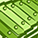# App Development

Discussion board where members can learn more about Qlik Sense App Development and Usage.

Announcements
Welcome to Qlik Community! Check out our new navigation! FIND OUT MORE
cancel
Showing results for
Did you mean:Creator

## Date Range in Set Analysis not working

Hello Everyone,

I have created 2 variables with below definition:

 Previous FY Start = Num(if(month(today())>=4,date('04/01/'&(year(today())-1),'MM/DD/YYYY'),date('04/01/'&(year(today())-2),'MM/DD/YYYY'))) Previous FY End = Num(if(month(today())>=4,date('03/31/'&(year(today())),'MM/DD/YYYY'),date('03/31/'&(year(today())-1),'MM/DD/YYYY')))

both the variables are returning correct values (1st Apr 2016 & 31st Mar 2017) in number format, but when I am using these variables in my Set I am not getting expected output, below is my Set expression:

 count({=\$(=Previous FY start)<=\$(=Previous FY End)"},[Status]*={'Closed'}>}[ID])

When I hard code the number values what I am getting from these variables then above Set expression works fine.

Not sure where I am going wrong, please help me solving this issue.

Regards,

Tauceef

Labels (1)
• ### expression

1 Solution

Accepted SolutionsMVP

Count({<DateNum = {">=\$(=\$(Previous FY start))<=\$(=\$(Previous FY End))"}, [Status] *= {'Closed'}>} [ID])

6 RepliesPartner - Champion III

Try these

count({<DateNum = {">=\$(Previous FY start) <=\$(Previous FY End)"}, [Status] *= {'Closed'}>} ID)

or

count({<DateNum = {">=\$(=Date(Previous FY start)) <=\$(=Date(Previous FY End))"}, [Status] *= {'Closed'}>} ID)

(assumes DateNum is in the default date format)

Logic will get you from a to b. Imagination will take you everywhere. - A EinsteinCreator
Author

Hi Jonathan,

1st Expression is returning 0 to me and 2nd one is returning same values which I am getting from the Set I have shared above.

with respect to DateNum values, these are correct only because as I have mentioned above, I am getting correct result when I hard code the numbers instead of Start date and End date variables.

Edited:

Just to add more if that helps: that filter is not getting applied i.e I am getting overall data as if no filter is applied, but when I replace variables with numbers it works fine.

Regards,

TauceefMVP

Count({<DateNum = {">=\$(=\$(Previous FY start))<=\$(=\$(Previous FY End))"}, [Status] *= {'Closed'}>} [ID])Creator
Author

Hi Sunny,

Thanks a lot man, it is working.

I actually changed my code yesterday to:

count({<[Status]*={'Closed'}>} if(DateNum>=\$(Previous FY start) and DateNum<=\$(Previous FY End),[ID]))

which worked for me, but your code is also working.

Would you be able to explain why it is like that(including extra \$()) because I have other variables as well which are working fine with the kind of code I have shared in my original post.

Thanks again.

Regards,

TauceefMVP

It all depends how your variable is defined.... I have found it difficult to explain when is it needed and when is it not needed...but these threads might help

The Magic of Dollar Expansions

The Magic of Variables

The Little Equals SignContributor IIITags
Community Browser